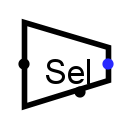#Bit Selector

 Library: Plexers Introduced: 2.0.5 Appearance:## Behavior

Given an input of several bits, this will divide it into several equal-sized groups (starting from the lowest-order bit) and output the group selected by the select input.

For example, if we have an eight-bit input 01010101, and we are to have a three-bit output, then group 0 will be the lowest-order three bits 101, group 1 will be the next three bits, 010, and group 2 will be the next three bits 001. (Any bits beyond the top are filled in with 0.) The select input will be a two-bit number that selects which of these three groups to output; if the select input is 3, then 000 will be the output. If the select input has an indeterminate or floating value (U) or error (E), the output bits will be in the indeterminate state (U). If one of the input data bits is indeterminate or floating (U) or error (E), then the corresponding output bit will be in the indeterminate state (U) or error (E).

## Pins (assuming component faces east)

West edge
Input: Data value from which bits should be selected for the output. Bit width matches Data Bits attribute.
East edge
Output: A group of bits from the data value, as selected by the select input. Bit width matches Output Bits attribute.
South edge
Input: Select input: Determines which of the bit groups should be routed to the output. Bit width is quotient of Data Bits and Output Bits, rounded up.

## Attributes

When the component is selected or being added, the digits 0 through 9 alter its Output Bits attribute, Alt-0 through Alt-9 alter its Data Bits attribute, and the arrow keys alter its Facing attribute.

Facing
The direction of the component (its output relative to input).
Data Bits
The bit width of the component's data input.
Output Bits
The bit width of the component's output.

None.

## Text Tool Behavior

None.

Back to Library Reference